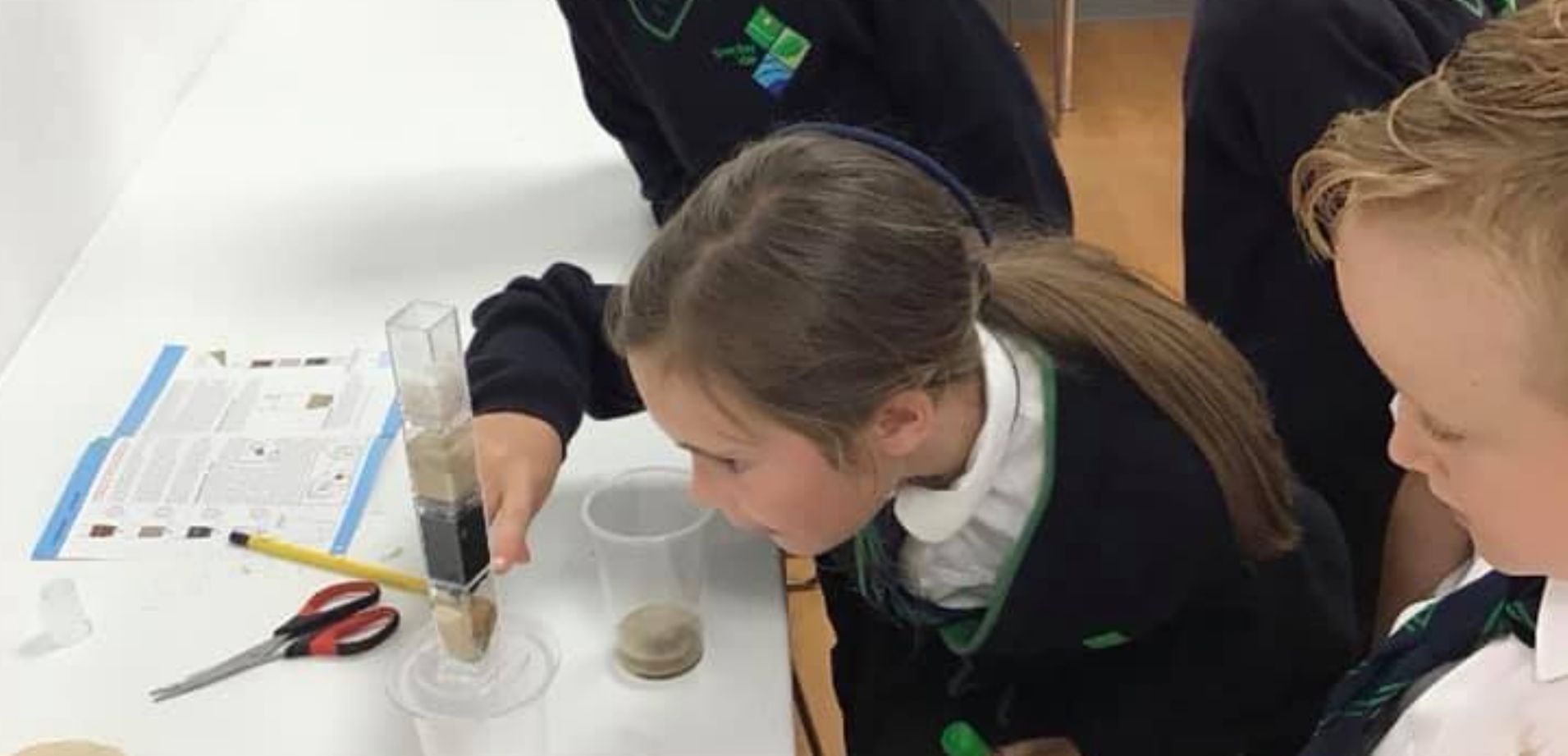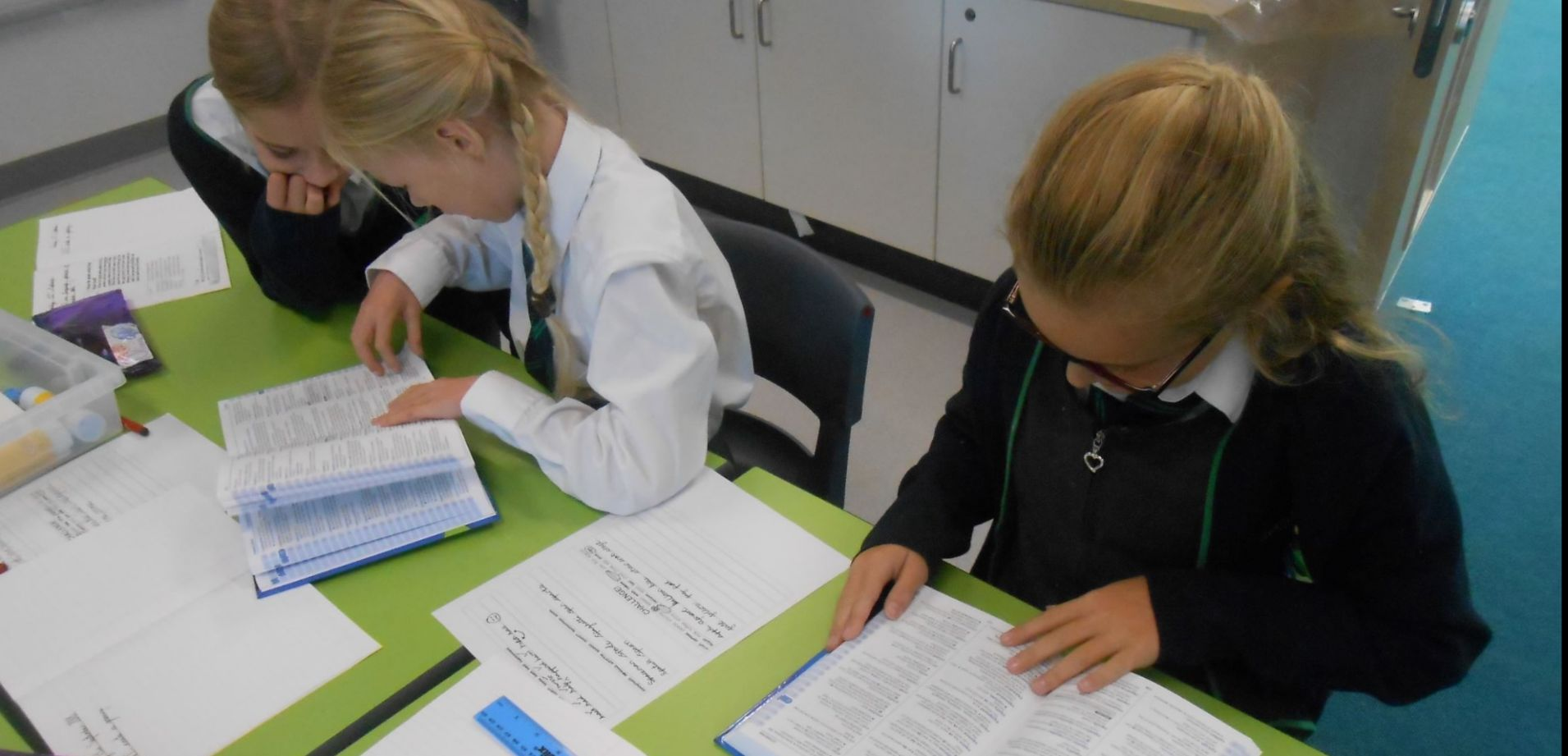Skip to content ↓
••••••••••••••••# Governor attendance at meetings

 250917 071117 160118 200218 130318 240418 220518 120618 160718 Cath Farrin X X X X X X X X X Sean Bruce X X X O O X X X O Tim Burton X X O O O X X X X Craig Moore X X X X X O X X O Amy Pearce X X X X X X X X X Helen Tipping X X X X X X X X X Rory Wakeling X X O X X O X X X Tom Brassil Appt X X X X X X X X Pat Skinner Appt O O X X X X

Appt - Appointed

X - Attended

O - Sanctioned absence